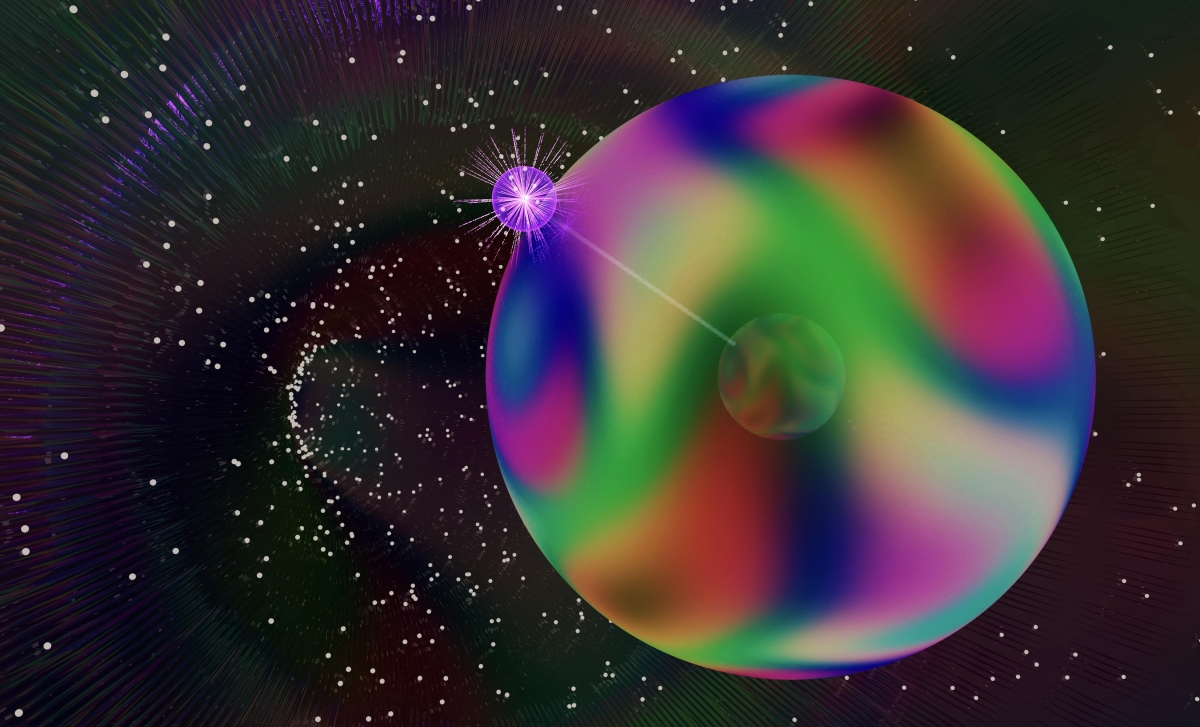# Second Chern number of a quantum-simulated non-Abelian Yang monopoleTopological order is often quantified in terms of Chern numbers, each of which classifies a topological singularity. Here, inspired by concepts from high-energy physics, we use quantum simulation based on the spin degrees of freedom of atomic Bose-Einstein condensates to characterize a singularity present in five-dimensional non-Abelian gauge theories—a Yang monopole. We quantify the monopole in terms of Chern numbers measured on enclosing manifolds: Whereas the well-known first Chern number vanishes, the second Chern number does not. By displacing the manifold, we induce and observe a topological transition, where the topology of the manifold changes to a trivial state.

Second Chern number of a quantum-simulated non-Abelian Yang monopole; S. Sugawa, F. Salces-Carcoba, A. R. Perry, Y. Yue, and I. B. Spielman; Science 360 1429–1434 (2018). doi:10.1126/science.aam9031

http://science.sciencemag.org/content/360/6396/1429